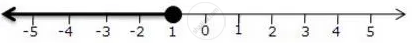Share

# For Graph Given Write an Inequation Taking X as the Variable - Mathematics

Course

#### Question

For graph given write an inequation taking x as the variable#### Solution

x <= -1, x in R

Is there an error in this question or solution?